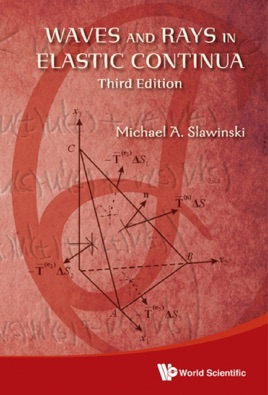• 529,00 kr

## Publisher Description

The present book — which is the third, significantly revised edition of the textbook originally published by Elsevier Science — emphasizes the interdependence of mathematical formulation and physical meaning in the description of seismic phenomena. Herein, we use aspects of continuum mechanics, wave theory and ray theory to explain phenomena resulting from the propagation of seismic waves.

The book is divided into three main sections: Elastic Continua, Waves and Rays and Variational Formulation of Rays. There is also a fourth part, which consists of appendices.

In Elastic Continua, we use continuum mechanics to describe the material through which seismic waves propagate, and to formulate a system of equations to study the behaviour of such a material. In Waves and Rays, we use these equations to identify the types of body waves propagating in elastic continua as well as to express their velocities and displacements in terms of the properties of these continua. To solve the equations of motion in anisotropic inhomogeneous continua, we invoke the concept of a ray. In Variational Formulation of Rays, we show that, in elastic continua, a ray is tantamount to a trajectory along which a seismic signal propagates in accordance with the variational principle of stationary traveltime. Consequently, many seismic problems in elastic continua can be conveniently formulated and solved using the calculus of variations. In the Appendices, we describe two mathematical concepts that are used in the book; namely, homogeneity of a function and Legendre's transformation. This section also contains a list of symbols.

Request Inspection Copy

Contents:Elastic Continua:DeformationsForces and Balance PrinciplesStress-Strain EquationsStrain EnergyMaterial SymmetryWaves and Rays:Equations of Motion: Isotropic Homogenous ContinuaEquations of Motion: Anisotropic Inhomogeneous ContinuaHamilton's Ray EquationsChristoffel's EquationsReflection and TransmissionLagrange's Ray EquationsVariational Formulation of Rays:Euler's EquationsVariational PrinciplesRay ParametersAppendices:Euler's Homogeneous-Function TheoremLegendre's TransformationList of Symbols
Readership: Theoretical seismologists, physicists and mathematicians interested in seismology.